Timescales: Absolute, Relative and Automatic

This example illustrates the various Timescale settings within the HDL Cosimulation block and explains how these affect the timing relationship of Simulink® and the HDL simulator. We use a simple Verilog parity check model to show the timing relationship of Simulink and the HDL simulator (ModelSim® or Incisive®) used for cosimulation.

Introduction

The model illustrates cosimulation of an HDL implementation of a simple parity checker and showcases the various options available in the Timescales pane of the HDL Cosimulation block. In that pane, Simulink time is related to HDL simulator time either in an absolute or relative sense. You can set the relationship explicitly or have the software automatically pick a relationship in either mode based on knowledge of the Simulink and HDL designs.

If you are using ModelSim or QuestaSim, the model parity_check_mq.slx should be open. If you are using Incisive or Xcelium, close the ModelSim model and open the model parity_check_in.slx.

% For ModelSim:
modelName = 'parity_check_mq';
open_system(modelName);
% For Incisive:
modelName = 'parity_check_in';
open_system(modelName);Verilog Code Used for Cosimulation

Parity checking is a method of adding a parity bit to a data stream in order to check that data for any errors. In this example we will use "even parity bit" scheme in which the parity bit is set to 1 if the number of ones in a given set of bits is odd (making the total number of ones, including the parity bit, even).

We select the input to the parity checker to be 8-bits and output as 1 bit. The Verilog code used for this example is paritychecker.v.

Preparing the Model for Cosimulation

In order to guide you through the various steps of this example, we have provided numbered steps which you can follow. Each numbered step is followed by a brief explanation of the same.

1. Launch ModelSim/Incisive

Before running the model, you must first launch the HDL simulator. Use the startup command provided within the model for this or run the simulator-specific command below. This also sets the HDL simulator resolution to 1ns.

For Modelsim/Questasim:

vsim('tclstart', paritycmds('1ns'));

For Incisive/Xcelium:

nclaunch('tclstart', paritycmds_in('1ns'));

2. Observe the settings within the HDL Cosimulation block's Ports pane

Double-click on the HDL Cosimulation block to edit the cosimulation parameters. Select the Ports pane.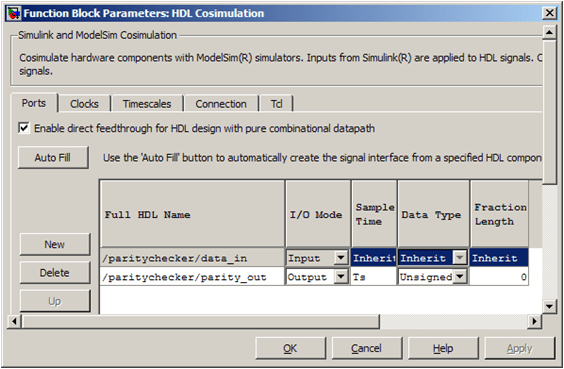• The sample time of the output signal is set to Ts - which is the sampling rate of the model. There are numerous ways to specify the value of Ts. We have set Ts from within the model's PreLoadFcn callback (see Create Model Callbacks (Simulink)). You can set the value of Ts in the base workspace at the MATLAB® Command prompt as well.

• Note that the option for allowing direct feedthrough has been checked - this is because our Verilog code is purely combinational.

This model is set up to work in three timing modes: Absolute, Relative, and Auto Timescale. You can read more about these modes in user guide section, Simulation Timescales.

Absolute Timescale

Absolute timing mode lets you define the timing relationship between Simulink and the HDL simulator in terms of absolute time units and a scale factor.

3. Click on the Absolute Mode annotation in the model to set the following:

• Sample time Ts = 10

• Set Timescales pane of HDL Cosimulation block to "1s in Simulink corresponds to 1ns in the HDL simulator"

Or invoke the following function:

paritytimescale('absolute');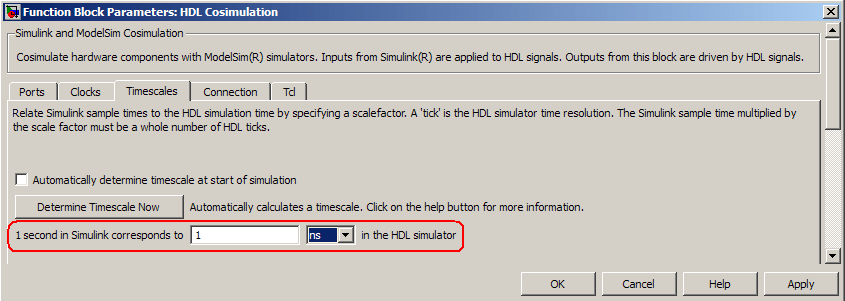The absolute time units in this case are 'ns' and the scale factor is '1'.

4. Run the model

The HDL simulator waveform shows the simulation run for 100ns, whereas the Simulink scope depicts how the model has run for 100s. This is expected for a Timescale setting of 1s in Simulink = 1ns in the HDL simulator.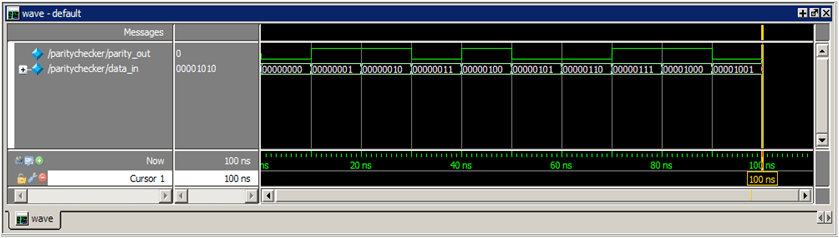Relative Timescale

Relative timing mode lets you define the timing relationship between Simulink and the HDL simulator in relative terms - that is, as some number of HDL simulator ticks.

5. Click on the Relative Mode annotation in the model to set the following:

• Sample time Ts = 10

• Set Timescales pane of HDL Cosimulation block to "1s in Simulink corresponds to 1 Tick in the HDL simulator":

paritytimescale('relative');The time units in this case are HDL simulator ticks and the scale factor is '1'.

6. Restart the HDL simulation.

For ModelSim: You can invoke a restart from within the HDL simulator.

vsim> restart

For Incisive: Close all HDL simulator windows first and relaunch the simulator.

nclaunch('tclstart',paritycmds_in('1ns'));

7. Run the model

The HDL simulator waveform shows the simulation run for 100ns, whereas the Simulink scope depicts how the model has run for 100s. This is expected for a Timescale setting of 1s in Simulink = 1 Tick in the HDL simulator (and the resolution of the simulator is set to 1ns). The waveforms obtained are similar to those we got in the absolute timescaling mode.

8. Change HDL Simulator resolution

Now we will see the effect of changing the HDL simulator resolution to 1ps. Close all of the HDL simulator windows and restart using a 1ps timescale resolution.

For Modelsim/Questasim:

vsim('tclstart',paritycmds('1ps'));

For Incisive/Xcelium:

nclaunch('tclstart',paritycmds_in('1ps'));

Run the Simulink model for the same 10 samples. The HDL simulator runs for 100ps since the Timescale is set to 1s in Simulink = 1 Tick in the HDL simulator.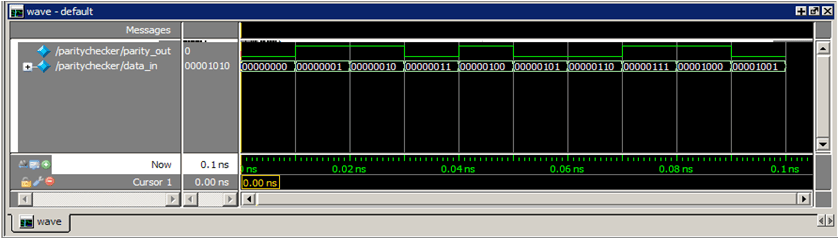Auto Timescale

Within the Timescales pane of the HDL Cosimulation block the Determine Timescale Now pushbutton calculates a timing relationship between Simulink and the HDL simulator. Please note that the link needs to find the resolution of the HDL simulator, and hence you will need to have the HDL simulator up and running.

Restart the HDL simulator using 1ns Resolution

Close all open HDL simulator windows first. Then:

For ModelSim:

vsim('tclstart',paritycmds('1ns'));

For Incisive:

nclaunch('tclstart',paritycmds_in('1ns'));

When you allow the link software to define the timing relationship, it attempts to set the timescale factor between the HDL simulator and Simulink to be as close as possible to 1 second in the HDL simulator = 1 second in Simulink (absolute timescale mode). If this setting is not possible, the HDL Verifier attempts to set the signal rate on the Simulink model port to the lowest possible number of HDL simulator ticks (relative timescale mode).

Now we will use the Auto Timescale within the model as shown in the next step:

9. Click on Determine Timescale Now button in the Timescales pane

This will suggest to set the Timescale to "1s in Simulink = 1e-10s in the HDL simulator". An info pane will be displayed: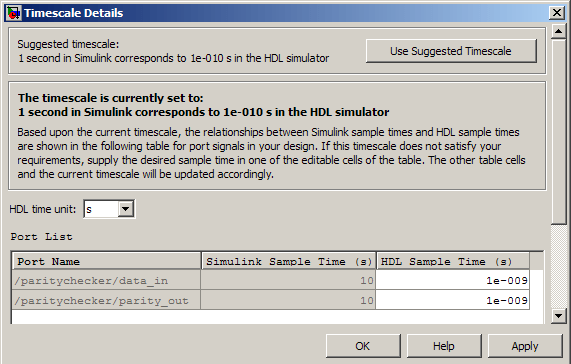The HDL Verifier software attempted to first achieve a 1:1 timescale setting, but failed to do so because the resolution of the HDL simulator is set to 1ns, and representing Ts=10 seconds would amount to 10e9 number of ticks which is greater than the 2^31 - 1 (=2.1475e9) allowable limit. The number 2^31 - 1 is the maximum value for an int32.

Once the HDL Verifier cannot establish an absolute 1:1 timescale it switches to relative timescale mode in which it will equate the fundamental sample time (Ts=10s) with 1 HDL tick. Hence 10s in Simulink will correspond to 1 HDL tick, that is, 1s in Simulink corresponds to 0.1 Tick in the HDL simulator.

Apply the changes suggested by the HDL Cosimulation block.

11. Run the model

The waveforms within the HDL simulator and from the Simulink scope are shown below. Note how the HDL simulator progresses through 10ns whereas the Simulink model runs for 100s:It is important to understand why the HDL Verifier did not choose a 1:1 mapping. If you were to set the Timescales setting to "1s in Simulink corresponds to 1s in the HDL simulator", apply this change, and run the model, Simulink will give you the following error: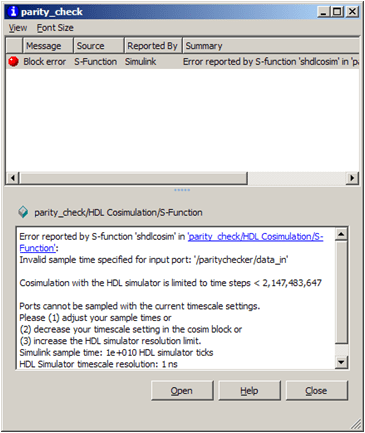12. Set an irrational sampling time (Ts = pi*1e-9)

If you were to use irrational sample times within Simulink, for example, Ts = pi*1e-9, the Auto Timescale will not be able to maintain an exact relationship between Simulink simulation and HDL simulation time. In this case, although the results of cosimulation are correct, the time axes of the Simulink scope and HDL simulator will not have a 1:1 relation.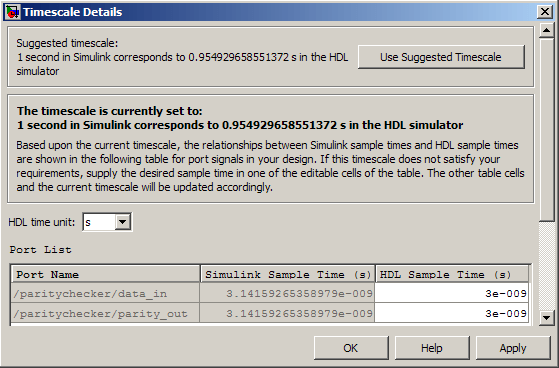13. Set Timescale to '1s in Simulink = 1s in HDL simulator'

In order to understand why 1:1 relationship is not possible, set the Timescale to '1s in Simulink = 1s in HDL simulator', and run the model (after restarting the simulation in the HDL simulator). A 1:1 timing relationship is not possible in this case as pi is an irrational number and is not an integral multiple of the 1ns (the HDL simulator resolution). Simulink will give you an error message in this case, as shown in the following image:Note: Each time you change the Timescale setting within the HDL Cosimulation block, you will need to restart the simulation run within the HDL simulator.

HDL Verifier DocumentationGet trial now